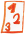Custom math worksheets at your fingertips# Details for problem "Shade fractions"

Quickname: 8211

## Summary

An image of s subdivided shape is shown. Shade according to given fraction.

## Example## Description

A shape is divided into a number of even-sized pieces. The number of pieces constitutes the denominator of a fraction.

A fraction is given; the respective fraction of the shape has to be shaded. It can be chosen that the given fraction's denominator exactly matches the number of pieces of the shape, or that it may be necessary to reduce the fraction first, or that it may be necessary to expand the fraction appropriately.

If expansion or reduction may be required, there is an option that a hint may be given in the task description.

The number of problems can be selected.

Download free worksheets for this math problem here. The worksheet contains the problems only, the solutions sheet includes the answers. Just click on the respective link.

•Worksheet 1Solution sheet with answers
•Worksheet 2Solution sheet with answers
•Worksheet 3Solution sheet with answers

If you can not see the solution sheets for download, they may be filtered out by an ad blocker that you may have installed. If this is the case, please allow ads for this page and reload the page. The solution sheets will then reappear.

• Do the sample worksheets do not really fit?
• Do you need more math worksheets, with a different level of difficulty?
• Would you like to combine different problems on a worksheet and adjust them to your needs?
• As a teacher, you can put together your own worksheets using the automatically generated math problems provided.
With a free initial credit, you can start creating your own math worksheets in a few minutes.

It does not cost anything to try! Register here, to create custom worksheets now!

## Customization options for this problem

Parameter
Possible values
Number of problems
1, 2, 3, 4, 5, 6, 7, 8, 9, 10
Denominator...
matches, reduce if necessary, expand if necessary, reduce/expand as needed
Hint on reduction/expansion
Yes, No

Remark
Description